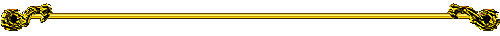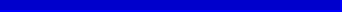and his Conjectures

```
Here's an "oldie but goodie" for you this time!Some time long ago a mathematician, named C. Goldbach, was playing
around with prime numbers and noted the following oddity:

All even numbers, greater than 2, can be expressed as
the sum of two primes.

This is really a simple idea, as these few examples will show:

20 = 7 + 13	    34 = 3 + 31	        42 = 13 + 29

66 = 19 + 47	   72 = 11 + 61	   100 = 47 + 53

So what's so hard about that, you ask?  Well, just try proving that it is true
for ALL even whole numbers!  There's the rub.  Ever since Goldbach made this
observation, mathematicians, professional and amateur alike, have tried to do
that.  But with no real success.

And that's why it is called a "conjecture", my friends.

A conjecture is a statement that you think is probably true, at least based
on all the information at hand.  But a "for sure" proof is not available.  You see,
it is not enough, as in a case like the one before us, to just say, "See!  All the
even numbers I've tested so far can all be expressed as the sum of a pair of primes.
So all of them must behave the same way."  Mathematics doesn't work that way.So where do we go from here, you ask?  Well, one of my favorite activities
for my students is to ask the question:

How many pairs of primes can be found
for any specific given even number?

This can be shown quickly by an example: 24.

5 + 19		7 + 17		11 + 13

So 24 can be expressed with three pairs of primes.

For those interested in making this investigation a bit more elegant, we can
define this with function notation of advanced algebra in the following way:

Let GN(n), the "Goldbach Number of n",
be defined as the number of pairs of primes
the sums of which are the number n.

So for our example, we would write

GN(24) = 3.Now, it's easy to see that this is a good function, as every n (input) has a
single GN(n) (output).  But what is not so obvious is this fact: as the value of n
increases, the same can not always be said for GN(n).  Here's a specific
example of what I am saying here:

24 < 26		but GN(24) = GN(26)

because GN(26) also equals 3.  [3+23, 7+19, and 13+13]

And even more significant is the case for n = 26 and n = 28.  Observe:

26 < 28		but GN(26) > GN(28)

because GN(28) is only 2.  [5+23 and 11+17]  And there are many more such
instances of this phenomenon.  How many can you find?

Speaking in the language of algebra, we could say:

If n < n+k for some positive integer k,
it is not always true that

GN(n)  <  GN(n+k).

Find the GN values for all the even numbers from 4 to 100.

To assist you with your work here, a nice table of all the primes between
1 and 1000 can be found by clicking HERE.Extension

Why stop with pairs of primes and the even numbers?  Let's also use trios
of primes and go for the odd numbers.  Mr. Goldbach did, or so I hear.

You surely will find plenty to challenge you here.

```